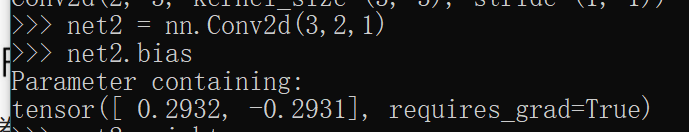# 多输入多输出通道

2 Likes

1、卷积操作看成是提取特征的方式，卷积核神经元就是图像处理中的滤波器，卷积层的每个滤波器都会有自己所关注一个图像特征，比如垂直边缘，水平边缘，颜色，纹理等等，这些所有神经元加起来就好比就是整张图像的特征提取器集合。这种提取特征方式与位置无关（就如why-conv里说的平移不变性：不管检测对象出现在图像中的哪个位置，神经网络的前面几层应该对相同的图像区域具有相似的反应，即为“平移不变性”）。个人理解这种不变性是基于物理实际的，即相同的特征模式对应于一种物理实际，比如物体间的边缘界面理论上是可以通过空间图像的差异区分（体现在图像颜色上就是色差值和色差梯度等这些指标，当然前提是图像有足够高的锐度），那么这种提取后的特征也应该适用于图像的其它部分。所以对图像的各个输入，单个二维卷积张量，也就是文中的一个Kh*kw矩阵是权值共享的，偏置项也是如此。
2、针对“每个卷积核里的每个参数共用一个偏差吗”，我觉得是的，共用一个偏置bias（你说的偏差）即可。因为互相关运算对偏置项是求和运算，即使你给每个参数设置不同的偏置值，最终这些偏差求和后也只会得到一个偏置值。而之所以能对图像不同部分应用同一个偏置值，原因是上面第一条。
3、你说的“感觉和多层感知机里的每个输入都有独立的偏差不太一样”的说法应该不对。偏置bias（你说的偏差）是针对输出而言的，不是针对输入，偏置项的维度跟输出项的维度是一样的，而不是跟输入项，不是“每个输入都有独立的偏差"，而是每个输出维度都有独立的偏差（当然很多时候，bias初始值在各个维度上的值都设为一样）。

4 Likes

# 6.4.5 练习

1. 没理解题意，不懂两个卷积核的结构是什么样的。。。

2. 2.1 ⌊(𝑛ℎ−𝑘ℎ+𝑝ℎ+𝑠ℎ)/𝑠ℎ⌋×⌊(𝑛𝑤−𝑘𝑤+𝑝𝑤+𝑠𝑤)/𝑠𝑤⌋×ci×co
2.2 ci×h×w+co×ci×kh×kw+co×⌊(𝑛ℎ−𝑘ℎ+𝑝ℎ+𝑠ℎ)/𝑠ℎ⌋×⌊(𝑛𝑤−𝑘𝑤+𝑝𝑤+𝑠𝑤)/𝑠𝑤⌋
2.3 co×ci×kh×kw
2.4 ⌈kℎ/sℎ⌉×⌈kw/sw⌉×co×ci×kh×kw

3. 4倍。增加(ph×pw)/(sh×sw)×ci×co的计算数量

4. h×w×ci×co

5. 因为输入的X和K是浮点数，后续浮点数计算会有误差

def corr2d_matmul(X, K):  #@save
"""计算二维互相关运算。"""
h, w = K.shape
yh, yw = (X.shape - h + 1, X.shape - w + 1)
K = K.reshape((h * w, 1))
Y = []
for i in range(yh):
for j in range(yw):
Y.append(X[i:i + h, j:j + w].reshape(h * w))
Y = torch.stack(Y, 0)
Y = torch.matmul(Y, K).reshape(yh, yw)
return Y

def corr2d_multi_in_matmul(X, K):
# 先遍历 “X” 和 “K” 的第0个维度（通道维度），再把它们加在一起
return sum(corr2d_matmul(x, k) for x, k in zip(X, K))

def corr2d_multi_in_out_matmul(X, K):
# 迭代“K”的第0个维度，每次都对输入“X”执行互相关运算。
# 最后将所有结果都叠加在一起

1 Like

4.1.2，维度为（k1H+K2H-1）X（K1W+K2W-1）
4.1.3，反之不一定1 Like

2.1 计算成本:
1.kernel 每次滑动要进行kh * kw次乘积运算以及kh * kw - 1次加法运算. 一共有ci层所以是ci * kh * kw + ci * kh * kw - 1
2.输出图像尺寸(h-kh+2ph)/sh + 1以及（w-kw+2pw)/sw + 1
3.一共co个核，计算成本为(ci * kh * kw + ci * kh * kw - 1) * ((h-kh+2ph)/sh + 1) * ((h-kh+2ph)/sh + 1) * co

1 Like
1. 应该是要证明两个较小的卷积层能够使用一个较大的卷积层来代替。
1.1 证明的话通过最后感受野的大小一致可以推导出来。
1.3 反之应该也可以。
2. 假设单通道最后输出的维度是mh和mw
2.1 单通道卷积核计算一次出进行kh * kw次乘法以及kh * kw - 1次加法，需要遍历mh * mw次。多个输入通道之间需要相加，一共是mh * mw * (ci - 1)次。所以最后计算成本是 co * [(mh * mw) * (kh * kw * 2 -1) + mh * mw * (ci - 1)]
2.2 内存包括输入、输出、卷积核的大小(没有算梯度的)。ci×h×w+co×ci×kh×kw+co×mh×mw。
1 Like1 Like

Q1: 假设我们有两个卷积核，大小分别为k_1和k_2（中间没有非线性激活函数）。
1. 证明运算可以用单次卷积来表示。

2. 这个等效的单个卷积核的维数是多少呢？

3. 反之亦然吗？

Q2: 假设输入为c_i×h×w，卷积核大小为c_o×c_i×k_h×k_w，填充为(p_h, p_w)，步幅为(s_h, s_w)。
1. 前向传播的计算成本（乘法和加法）是多少？

2. 内存占用是多少？（是指参数所占的内存？还是指参数以及数据的内存占用？）

3. 反向传播的内存占用是多少？

4. 反向传播的计算成本是多少？

Q3: 如果我们将输入通道c_i和输出通道c_o的数量加倍，计算数量会增加多少？如果我们把填充数量翻一番会怎么样？
A3: 如果输入通道c_i和输出通道c_o的数量加倍，则计算量变为原来的4倍；如果填充数量翻一番，则计算量增加c_o×c_i×(p_w/s_w)×(p_h/s_h)。

Q4: 如果卷积核的高度k_h=k_w=1和宽度是，前向传播的计算复杂度是多少？
A4: c_o×(2×c_i-1)×(h×w)

Q5: 本节最后一个示例中的变量Y1和Y2是否完全相同？为什么？

Q6: 当卷积窗口不是1×1时，如何使用矩阵乘法实现卷积？
A6:

def corr2d_multi(X, K):
h, w = K.shape
outh = X.shape - h + 1
outw = X.shape - w + 1
K = K.reshape(-1, 1)
Y = []
for i in range(outh):
for j in range(outw):
Y.append(X[i:i + h, j:j + w].reshape(-1))
# 这个完全没想到，感谢@thirty
Y = torch.stack(Y, 0)
# 用矩阵乘法表示互相关运算
res = (torch.matmul(Y, K)).reshape(outh, outw)
return res

def corr2d_multi_in(X, K):
"""多输入通道"""
# 先遍历“X”和“K”的第0个维度（通道维度），再把它们加在一起
# return sum(d2l.corr2d(x, k) for x, k in zip(X, K))
return sum(corr2d_multi(x, k) for x, k in zip(X, K))

def corr2d_multi_in_out(X, K):
"""多输出通道"""
# 迭代“K”的第0个维度，每次都对输入“X”执行互相关运算。
# 最后将所有结果都叠加在一起

3 Likes
def conv2d_mm(x,k):
'''使用im2col方式计算，没有考虑batch size'''
#计算输出的尺寸
h, w = k.shape[-2], k.shape[-1]
outh = x.shape[-2] - k.shape[-2] + 1
outw = x.shape[-1] - k.shape[-1] + 1
#摘取数据
tmpx = []
for i in range(outh):
for j in range(outw):
tmpx.append(x[:,i:i+h, j:j+w].reshape(-1,1))
#把摘取的数据堆叠为矩阵，每一列为相应位置摘取的所有通道的数据，用来与卷积核做矩阵乘法
#使用torch.cat()和torch.stack()都可以
tmpx = torch.cat(tmpx, 1)
#tmpx = torch.stack(tmpx, 1).squeeze()
#卷积核变为行向量，每一行是一个卷积核的参数，共有c_o行
k = k.reshape(k.shape, -1)
#把结果reshape为c_0 X outh X outw形状
y=torch.mm(k, tmpx).reshape(k.shape, outh, outw)
return y

1. 请问一下Q2第一问可以写一下推导过程么（答案都可以稍微写下推导过程，方便我们理解你的思路并验证），比如乘法计算量为
c_o×c_i×(⌊(ℎ−𝑘_ℎ+𝑝_ℎ+𝑠_ℎ)/𝑠_ℎ⌋×⌊(𝑤−𝑘_𝑤+𝑝_𝑤+𝑠_𝑤)/𝑠_𝑤⌋)×(k_h×k_w)
加法计算量为（我理解是因为你考虑到n个数只要相加n-1次）
c_o×c_i×(⌊(ℎ−𝑘_ℎ+𝑝_ℎ+𝑠_ℎ)/𝑠_ℎ⌋×⌊(𝑤−𝑘_𝑤+𝑝_𝑤+𝑠_𝑤)/𝑠_𝑤⌋)×(k_h×k_w-1)
在李沐老师的B站视频中，算法复杂度好像可以直接用O(Ci * Co * Kh * Kw * Mh * Mw)
其中
输入x: Ci * Nh * Nw
核W: Co * Ci * Kh * Kw
偏差B: Co * Ci
输出Y: Co * Mh * Mw
我理解就算是按照你的结果，化简一下就是
c_o×c_i×(⌊(ℎ−𝑘_ℎ+𝑝_ℎ+𝑠_ℎ)/𝑠_ℎ⌋×⌊(𝑤−𝑘_𝑤+𝑝_𝑤+𝑠_𝑤)/𝑠_𝑤⌋)×(2×k_h×2×k_w-1)
在计算复杂度表达中，可以省去常数项，应该也可以表示为
c_o×c_i×(⌊(ℎ−𝑘_ℎ+𝑝_ℎ+𝑠_ℎ)/𝑠_ℎ⌋×⌊(𝑤−𝑘_𝑤+𝑝_𝑤+𝑠_𝑤)/𝑠_𝑤⌋)×(k_h×k_w)
同李沐老师给出的公式
如果有任何错误，请指正
1 Like

2.Q3的A3中，填充数量翻一番，计算量增加是怎么计算的呢？

$$(n_h-k_h+p_h+1)\times(n_w-k_w+p_w+1)$$

1. Q6我想补充一点细节，之前我看到这个代码是懵的，因为没有示例不够直观，我添加了一些示例和代码注释，应该可以帮到和我一样有类似困惑的人，代码如下：
def corr2d_multi(X,K):
h,w=K.shape
outh=X.shape-h+1
outw=X.shape-w+1
K=K.reshape(-1,1)
Y=[]
for i in range(outh):
for j in range(outw):
Y.append(X[i:i+h,j:j+w].reshape(-1))#将需要计算的局部矩阵拉伸成一个向量并纳入Y
Y=torch.stack(Y,0)
res=torch.matmul(Y,K).reshape(outh,outw)
return res

def corr2d_multi_in(X, K):
# 先遍历“X”和“K”的第0个维度（通道维度），再把它们加在一起
return sum(corr2d_multi(x, k) for x, k in zip(X, K))

def corr2d_multi_in_out(X, K):
# 迭代“K”的第0个维度，每次都对输入“X”执行互相关运算。
# 最后将所有结果都叠加在一起

X = torch.tensor([[[0.0, 1.0, 2.0], [3.0, 4.0, 5.0], [6.0, 7.0, 8.0]],
[[1.0, 2.0, 3.0], [4.0, 5.0, 6.0], [7.0, 8.0, 9.0]]])
K = torch.tensor([[[0.0, 1.0], [2.0, 3.0]], [[1.0, 2.0], [3.0, 4.0]]])
K = torch.stack((K, K + 1, K + 2), 0)
print(X.shape)#torch.Size([2, 3, 3])
print(K.shape)#torch.Size([3, 2, 2, 2])
print(corr2d_multi_in_out(X, K).shape)#torch.Size([3, 2, 2])
#上面的输出中3=K.shape,[2,2]为输出层大小,具体计算公式为上一章的6.3.1,Xh-Kh+1=3-2+1=2,w同理


1.a 这个题目有点坑，应该是两个卷积核，一个在前，一个在后，而不是并行，那么最后的feature map大小为：
n-K1+1-K2+1=n-(K1+K2-1)+1

1.b 卷积核大小为K1+K2-1
1.c 同样的，一个卷积核也可以逆过来使用两个卷积核进行替换，如一个55可以替换成两个33